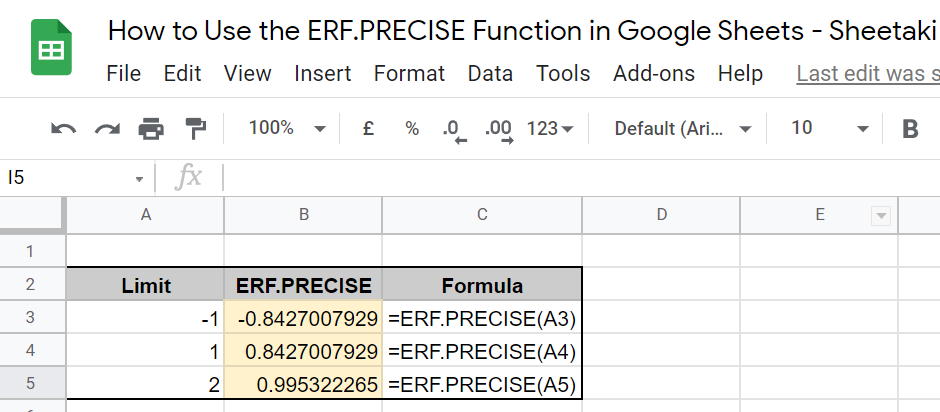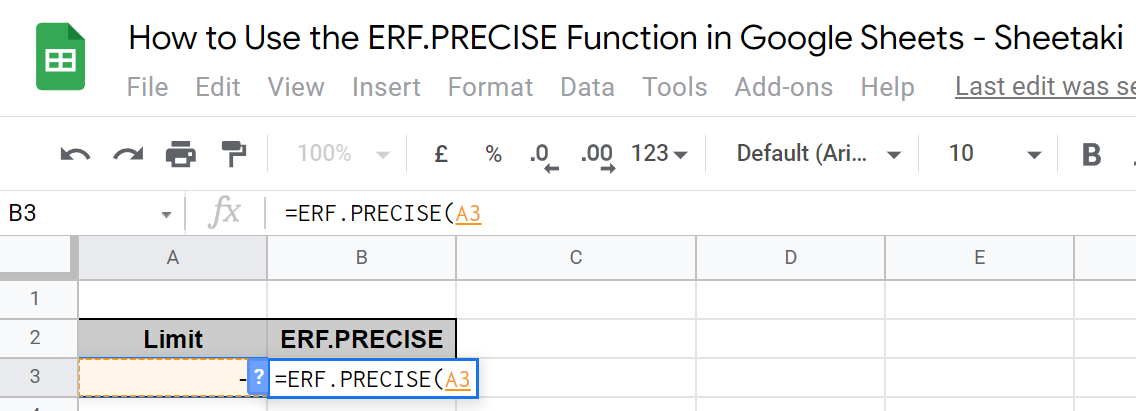# How to Use the ERF.PRECISE Function in Google Sheets

The `ERF.PRECISE` function in Google Sheets calculates for the Gauss error function integrated between 0 and a given limit.

Just like the `ERF` function, the number generated represents how well your model fits the data and a lower number means you have less error. The main difference is that you can provide both upper and lower limits in the `ERF` function, while the `ERF.PRECISE` function necessitates 0 as one limit.

`ERF.PRECISE` is defined as follows:For a random variable normally distributed with a mean of 0 and a variance of 1/√2, ERF(x) describes the probability of the variable falling in the given range [0,x].

This basically works the same way as the `ERF` function, so check out our article on how to use the ERF function in Google Sheets to see if that’s a better use for you.

Let’s get to learning how to write the `ERF.PRECISE` function in Google Sheets.

## The Anatomy of the ERF.PRECISE Function in Google Sheets

The syntax (the way we write) of the ERF.PRECISE function goes as follows:

`=ERF.PRECISE(limit)`

Let’s dissect this thing and understand what each of these terms means:

• = the equal sign is just how we start any function in Google Sheets.
• ERF.PRECISE () is our Gauss error function.
• limit is the lower or upper boundary for the integral.

Note that you will get the #VALUE! error value if your limit is non-numeric. Also, you can use both positive and negative integers as your given limit.

## Real Example of Using the ERF.PRECISE Function in Google Sheets

Now, let’s take a look at some numbers I’m presenting to see how an ERT.PRECISE function will look like in Google Sheets.In the table, the function calculates the Gaussian error integral value for the limit as -1, then 1, and then 2. Besides the negative and positive signs, the result should be the same for (1,0) and (-1,0) since their distance on a distribution table is the same.

At this point, I’ll show you how you can do this yourself with comprehensive steps.

## How to Use the ERF.PRECISE Function in Google Sheets

1. First, select the cell where you wish to put the result of your `ERF.PRECISE` function. In this case, that’s B3.2. Next, enter the equal sign ‘=’ to begin the function. Then, follow it with the name of the function, which is ‘`ERF.PRECISE`’ (or ‘`erf.precise`’, both should work). While typing that down, an auto-suggest box should appear with the different variations of the `ERF` function. Click on the `ERF.PRECISE` option.3. Now, you can read the pop-out with more information about the function. Google Sheets will show you the same information it shows for the `ERF` function.4. Proceed to enter the number that serves as your limit. In this case, we select the cell where the data is entered prior, which is A3.5. Then, simply enter a close parenthesis ‘)’ and select Enter on your keyboard.That’s it! You’ve just learned how to use the `ERF.PRECISE` function in Google Sheets.

What is the difference between `ERF` and `ERF.PRECISE`?

In an `ERF` function, you can provide both upper and lower limits. On the other hand, 0 is the default pair of your `ERF.PRECISE` limit. Note that an `ERF` function can technically serve as an `ERF.PRECISE` function since its upper_bound parameter is not mandatory.

What is `ERFC.PRECISE`?

`ERFC` stands for ‘complementary error function’ and gives out the value of 1.0 -erf(x).

`ERFC.PRECISE` calculated for the complementary error function integrated between a given lower limit and infinity.

How do I use `ERF.PRECISE` in Excel?

`ERF.PRECISE` is used the same in Google Sheets and Excel. Simply follow the instructions in this article to use the function in Excel. Note that while the `ERF` function has been available since before the 2010 update of Excel, that is not the same for `ERF.PRECISE`

##### You May Also Like## How to Use WORKDAY.INTL Function in Google Sheets

The WORKDAY.INTL function in Google Sheets is useful if you want to get a date that is the…## How to Auto-Populate Dates Between Two Given Dates in Google Sheets

To auto-populate dates between two given dates in Google Sheets is useful if you have a start date…## How To Use CHIDIST Function in Google Sheets

The CHIDIST function in Google Sheets is useful when you need to calculate the right-tailed chi-squared distribution of…## How to Use ARRAY_CONSTRAIN Function in Google Sheets

The ARRAY_CONSTRAIN function in Google Sheets is useful for situations where you need to extract a portion of…